# Functions Set 1

Go back to  'SOLVED EXAMPLES'

Example - 1

Find the domain for f(x) = \begin{align}\frac{1}{{\left[ {|x - 1|} \right] + \left[ {|5 - x|} \right] - 4}}\end{align}

Solution: The denominator is a bit complicated and we need to analyse it in detail to determine where it can become zero.

The fastest and easiest way would be to visualise the graph. Draw the graphs for | x – 1 | and | 5 – x |, apply the greatest integer function on these graphs separately, then add them and find the values of x for which this sum becomes 4. For these values of x, the denominator of f(x) becomes 0.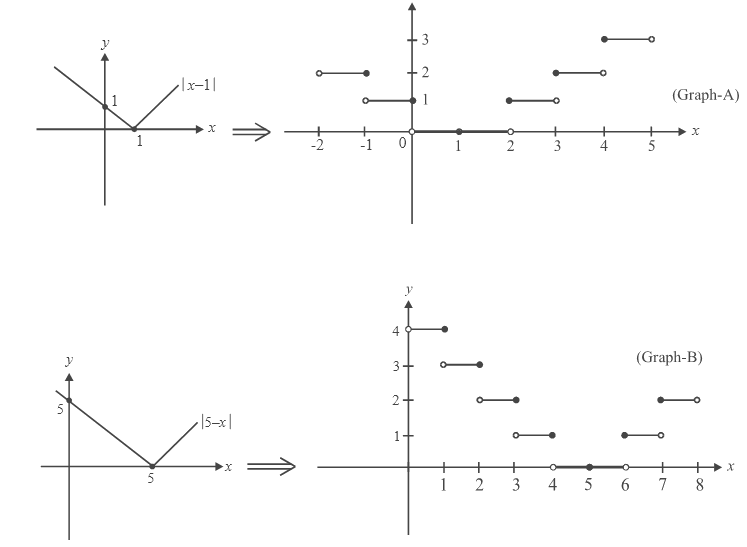Now add the graphs of A and B point by point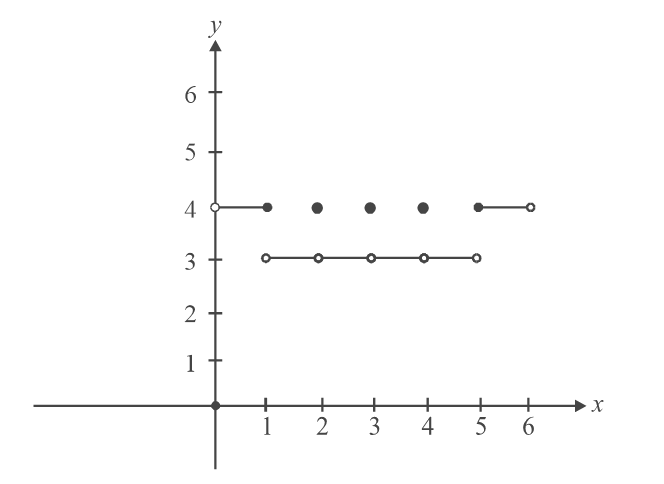We see that the value of (graphs A + graph B) is 4 for the following values of

$$x:\left( {0,1],\{ 2,3,4\} ,[5,6} \right)$$ .    Hence, D = $$\mathbb{R}\backslash \{ (0,\,\,1],\,\,2,\,\,3,\,\,4,\,\,[5,\,\,6)\}$$

Example - 2

Draw the graphs for:

(a)   $$y = \sqrt {\{ x\} } ,\;\;\;|y| = \sqrt {\{ x\} }$$

(b)   $$y = [x] + \sqrt {\{ x\} }$$

(c)    $$y = - [x] + \sqrt {\{ x\} }$$

Solution: Let us first draw the graph for $$y = \sqrt {\{ x\} }$$  . Now, {x} is the same as x for 0 < x < 1. In this interval,  $$\sqrt {\{ x\} }$$ will be the same as $$\sqrt x$$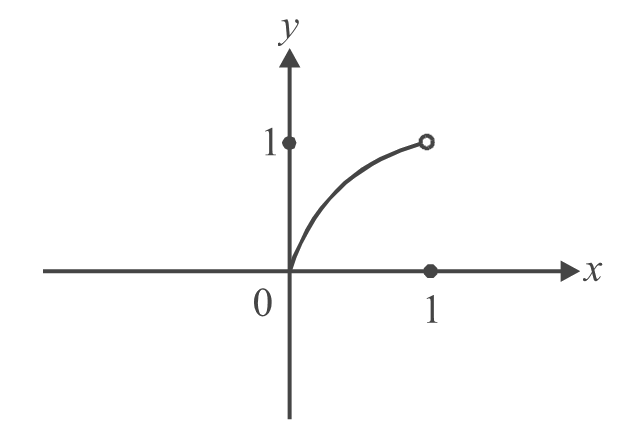We can easily see that this same curve will be repeated in every previous and subsequent unit interval, since {x} is the same in all such intervals. Hence we obtain the graph of $$y = \sqrt {\{ x\} }$$

(a)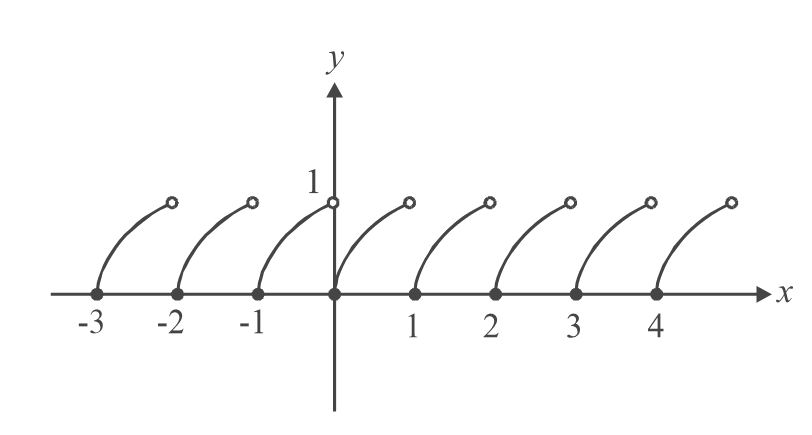Now we can easily draw $$|y| = \sqrt {\{ x\} }$$  by taking a reflection in the x-axis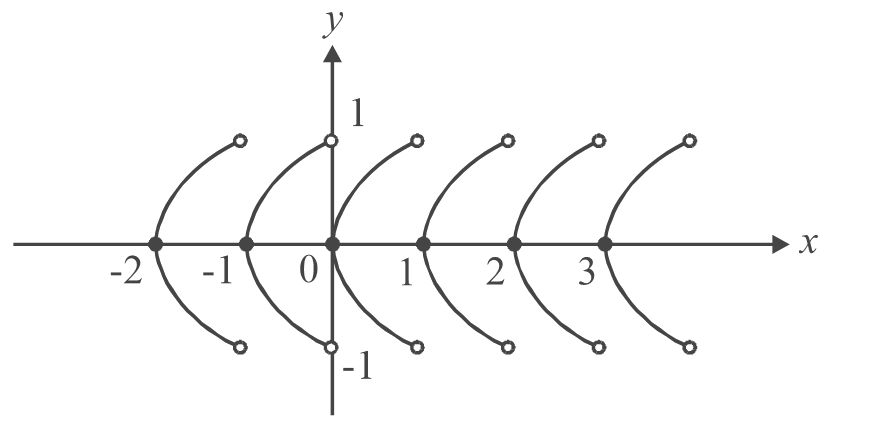(b)  In any interval $$n \le x < n + 1$$  (where n is an integer), [x] has the value n. In any such interval therefore,  the graph of  $$y = [x] + \sqrt {\{ x\} }$$ will be the graph of  $$\sqrt {\{ x\} }$$  + integer n. This will be the graph segment of  $$\sqrt {\{ x\} }$$  lifted by n units.

For example.

$0 \le {\rm{}}x < 1{\rm{}}\quad \Rightarrow \quad {\rm{ }}y = \sqrt {\{ x\} }$

$1 \le x < 2{\rm{ }}\quad \Rightarrow \quad y = 1 + \sqrt {\{ x\} }$

$- 1 \le x < 0\quad \Rightarrow \quad y = - 1 + \sqrt {\{ x\} }$

The graph is drawn below.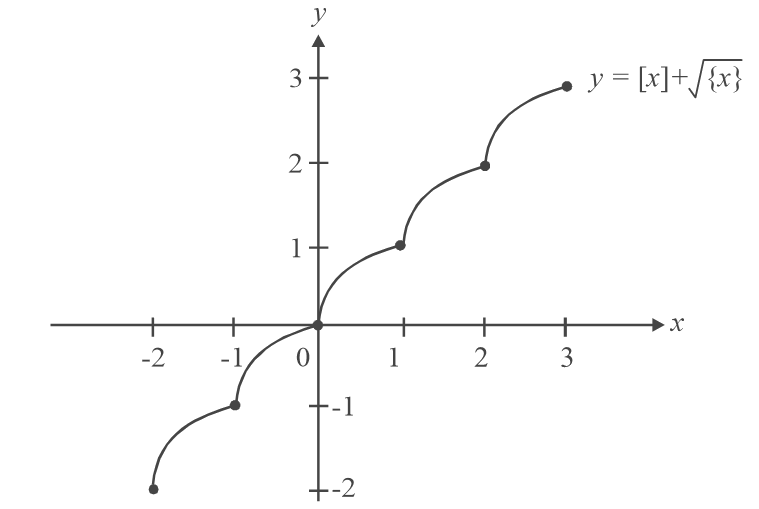Note that this graph has no holes (or breaks) now.  We express this fact by saying that this function is continuous. The function $$y = \sqrt {\{ x\} }$$ , is , on the other hand, discontinuous.

We will study continuity in more detail later.

(c)             On following the same lines as part (b), we obtain the graph of  $$y = - [x] + \sqrt {\{ x\} } .$$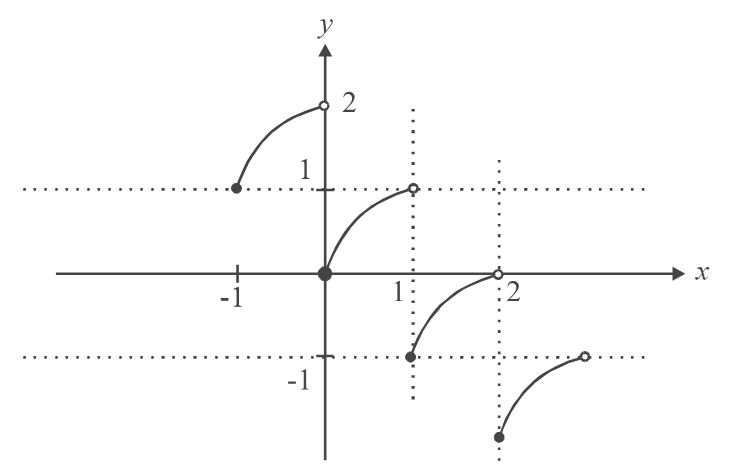This function, as we can see, is discontinuous.

Based on the discussions above, draw the graphs for $$y = \sqrt {\{ |x|\} }$$  ,

$y = \left| {[x] + \sqrt {\{ x\} } } \right|,\,\,\,|y| = \left| {[x] + \sqrt {\{ x\} } } \right|,\,\,\,|y| = [|x|] + \sqrt {|\{ x\} |}$

$y = [x] - \{ x\} \quad and \quad y = x + \{ x\}$

Learn from the best math teachers and top your exams

• Live one on one classroom and doubt clearing
• Practice worksheets in and after class for conceptual clarity
• Personalized curriculum to keep up with school